JEE  >  JEE Main Mathematics Mock - 1

# JEE Main Mathematics Mock - 1

Test Description

## 25 Questions MCQ Test JEE Main & Advanced Mock Test Series | JEE Main Mathematics Mock - 1

JEE Main Mathematics Mock - 1 for JEE 2023 is part of JEE Main & Advanced Mock Test Series preparation. The JEE Main Mathematics Mock - 1 questions and answers have been prepared according to the JEE exam syllabus.The JEE Main Mathematics Mock - 1 MCQs are made for JEE 2023 Exam. Find important definitions, questions, notes, meanings, examples, exercises, MCQs and online tests for JEE Main Mathematics Mock - 1 below.
Solutions of JEE Main Mathematics Mock - 1 questions in English are available as part of our JEE Main & Advanced Mock Test Series for JEE & JEE Main Mathematics Mock - 1 solutions in Hindi for JEE Main & Advanced Mock Test Series course. Download more important topics, notes, lectures and mock test series for JEE Exam by signing up for free. Attempt JEE Main Mathematics Mock - 1 | 25 questions in 60 minutes | Mock test for JEE preparation | Free important questions MCQ to study JEE Main & Advanced Mock Test Series for JEE Exam | Download free PDF with solutions
 1 Crore+ students have signed up on EduRev. Have you?
JEE Main Mathematics Mock - 1 - Question 1

### In the following question, a Statement of Assertion (A) is given followed by a corresponding Reason (R) just below it. Read the Statements carefully and mark the correct answer- Assertion(A):If Cr is the coefficient of xr in the expansion of (1 + x)20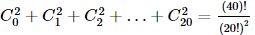Reason(R) : Cr = Cn − r for any positive integer n

JEE Main Mathematics Mock - 1 - Question 2

### The area (in square units) bounded by the curves y2 = 4x and x2 = 4y in the plane is

JEE Main Mathematics Mock - 1 - Question 3

### If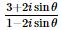sin θ is real, then θ =

JEE Main Mathematics Mock - 1 - Question 4

In the following question, a Statement of Assertion (A) is given followed by a corresponding Reason (R) just below it. Read the Statements carefully and mark the correct answer-
Assertion(A) :The inverse of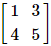does not exist.
Reason(R) :The matrix is non singular.

JEE Main Mathematics Mock - 1 - Question 5

If the line 3x-4y=λ touches the circle x2+y2-4x-8y-5=0, λ  can have the values

JEE Main Mathematics Mock - 1 - Question 6

The length of the tangent from (0,0) to the circle 2x2 + 2y2 + x - y + 5 = 0 is

JEE Main Mathematics Mock - 1 - Question 7

The differential equation which represents the family of plane curves y=exp. (cx) is

Detailed Solution for JEE Main Mathematics Mock - 1 - Question 7

y = ecx
dy/dx = c. ecx
y' = cy

JEE Main Mathematics Mock - 1 - Question 8

If sin y = x sin (a + y), then (dy/dx) =

JEE Main Mathematics Mock - 1 - Question 9

The fundamental period of the function f(x) = 2 cos 1/3(x - π) is

JEE Main Mathematics Mock - 1 - Question 10
If A, B, C are represented by 3 + 4i, 5 - 2i, -1 + 16i respectively, then A, B, C are
JEE Main Mathematics Mock - 1 - Question 11
Which of the following is not a statement ?
JEE Main Mathematics Mock - 1 - Question 12
In the following question, a Statement-1 is given followed by a corresponding Statement-2 just below it. Read the statements carefully and mark the correct answer-
Consider the planes 3x – 6y – 2z = 15 and 2x + y – 2z = 5.
Statement-1:
The parametric equations of the line of intersection of the given planes are
x = 3 + 14t, y = 1 + 2t, z = 15t.
Statement-2:
The vector 14î+2ĵ+15k̂ is parallel to the line of intersection of given planes
JEE Main Mathematics Mock - 1 - Question 13

In the following question, a Statement-1 is given followed by a corresponding Statement-2 just below it. Read the statements carefully and mark the correct answer-
Tangents are drawn from the point (17,7) to the circle x2+y2=169.
Statement-1:
The tangents are mutually perpendicular.
Statement-2:
The locus of the points from which mutually perpendicular tangents can be drawn to the given circle is x2+y2=338.

Detailed Solution for JEE Main Mathematics Mock - 1 - Question 13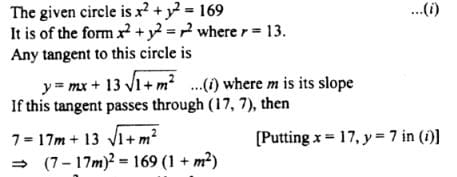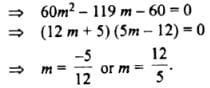Clearly, m1m2 = - 1.
Hence, the two tangents arc mutually perpendicular.
Statement 1 is true.
Now, the locus of the point of intersection of two mutually perpendicular tangents to the circle x2 + y2 = r2 is the director circle i.e. the circle x2 +y2 = 2r2
For the given circle r = 13. ..
Its director circle is x2 + y2 = 338.
Hence, statement 2 is true and  a cogect explanation of statement as the point (17, 7) lies on the director circle of the circle (i).

JEE Main Mathematics Mock - 1 - Question 14

The value of a for which the system of equations
a3x+(a+1)3y+(a+2)3z = 0
ax+(a+1)y+(a+2)z = 0
x+y+z = 0
has a non-zero solution, is

Detailed Solution for JEE Main Mathematics Mock - 1 - Question 14

The system of equation has a non-zero solution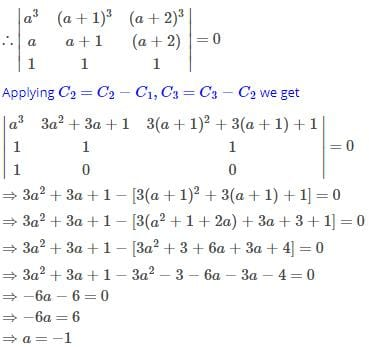JEE Main Mathematics Mock - 1 - Question 15

The pole of the line 2x + 3y − 4 = 0 with respect to the parabola y2 = 4 x is

JEE Main Mathematics Mock - 1 - Question 16

If nC12=nC8, then n=

Detailed Solution for JEE Main Mathematics Mock - 1 - Question 16JEE Main Mathematics Mock - 1 - Question 17

The chance of getting a doublet with 2 dice is

Detailed Solution for JEE Main Mathematics Mock - 1 - Question 17

Total outcomes = 36
Doublet are 6 (1,1),(2,2),(3,3),(4,4),(5,5),(6,6)

Probability of getting doublet = 6/36
= 1/6

JEE Main Mathematics Mock - 1 - Question 18

If cov. (x, y) = 0, then ρ(x, y) equals

Detailed Solution for JEE Main Mathematics Mock - 1 - Question 18

Covariance is a quantitative measure of the extent to which the deviation of one variable from its mean matches the deviation of the other from its mean. It is a mathematical relationship that is defined as:

Cov(X,Y) = E[(X − E[X])(Y − E[Y])]

Correlation between two random variables, ρ(X,Y) is the covariance of the two variables normalized by the variance of each variable. This normalization cancels the units out and normalizes the measure so that it is always in the range [0, 1]: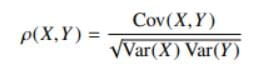JEE Main Mathematics Mock - 1 - Question 19

The two opposite vertices of a square on xy-plane are A(-1,1) and B(5,3), the equation of other diagonal (not passing through A and B) is

Detailed Solution for JEE Main Mathematics Mock - 1 - Question 19

Given: Here,AB is the diagonal of square.
The vertices of a square A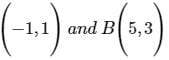Let the mid−point of AB be EThen coordinates of E are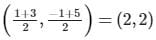Therefore equation of other diagonal is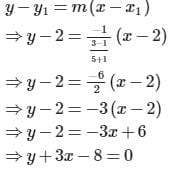JEE Main Mathematics Mock - 1 - Question 20

If the normal to the curve y=f(x) at the point (3,4) makes an angle 3π/4 with the positive x-axis, then f'(3)

Detailed Solution for JEE Main Mathematics Mock - 1 - Question 20

Given y = f(x)

differentiating w.r.t x

y' = f'(x) which is the slope of the tangent

Hence the slope of the normal is - 1/f'(x) = 3pi/4 = -1

therefore f'(x) = 1

Hence f'(3) = 1

*Answer can only contain numeric values
JEE Main Mathematics Mock - 1 - Question 21

Assume e-4/5 = 2/5. If x, y satisfy, y = ex and the minimum value of (x2 + y2) is expressed in the form of m/n then (2m - n)/5 equals (where m & n are coprime natural numbers)

Detailed Solution for JEE Main Mathematics Mock - 1 - Question 21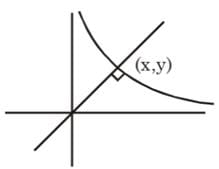OP2 = x2 + y2
y = ex, y' = ex,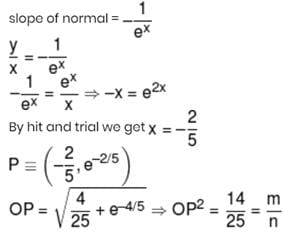*Answer can only contain numeric values
JEE Main Mathematics Mock - 1 - Question 22

Let ƒ(x) be non-constant thrice differentiable function defined on (–∞, ∞) such that ƒ(x) = ƒ(6 – x) and ƒ'(0) = 0 = ƒ'(2) = ƒ'(5). If 'n' is the minimum number of roots of (ƒ"(x))2 + ƒ'(x)ƒ"'(x) = 0 in the interval x ∈ [0, 6] then sum of digits of n equals

Detailed Solution for JEE Main Mathematics Mock - 1 - Question 22

ƒ(x) = ƒ(6 – x)
⇒ ƒ'(x) = –ƒ'(6 – x) .... (1)
put x = 0, 2, 5
ƒ'(0) = ƒ'(6) = ƒ'(2) = ƒ'(4) = ƒ'(5) = ƒ'(1) = 0
and from equation (1) we get ƒ'(3) = –ƒ'(3)
⇒ ƒ'(3) = 0
So ƒ'(x) = 0 has minimum 7 roots in
x ∈ [0, 6] ⇒ ƒ"(x) has min 6 roots in x ∈ [0,6]
h(x) = ƒ'(x).ƒ"(x)
h'(x) = (ƒ"(x))2 + ƒ'(x) ƒ"'(x)
h(x) = 0 has 13 roots in x ∈ [0, 6]
h'(x) = 0 has 12 roots in x ∈ [0, 6]

*Answer can only contain numeric values
JEE Main Mathematics Mock - 1 - Question 23

If the co-ordinate of the vertex of the parabola whose parametric equation is x = t2 – t + 1 and y = t2 + t + 1, t ∈ R is (a, b) then (2a + 4b) equals

Detailed Solution for JEE Main Mathematics Mock - 1 - Question 23

x = t2 – t + 1    .... (1)
y = t2 + t + 1    .... (2)
y – x = 2t  &  x + y = 2(t2 + 1)
________on elminating 't' we get
⇒ (x + y – 2) = 2(y - x)/22
(x – y)2 = 2(x + y – 2)
Axis : x – y = 0
Tangent at vertex : x + y – 2 = 0
Vertex : (1, 1) = (x, y)

*Answer can only contain numeric values
JEE Main Mathematics Mock - 1 - Question 24

If a, b, c, x, y, z are non-zero real numbers and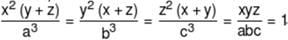then the value of (a3 + b3 + c3 + abc) equals

Detailed Solution for JEE Main Mathematics Mock - 1 - Question 24

x2(y + z)y2(z + x)z2(x + y) = a3b3c3 = x3y3z3
⇒ (x + y) (y + z) (z + x) = xyz
⇒ x2(y + z) + y2(z + y) + z2(x + y) + xyz = 0
⇒ a3 + b3 + c3 + abc = 0

*Answer can only contain numeric values
JEE Main Mathematics Mock - 1 - Question 25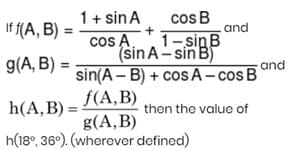Detailed Solution for JEE Main Mathematics Mock - 1 - Question 25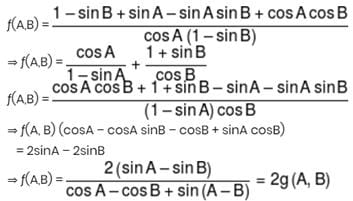## JEE Main & Advanced Mock Test Series

2 videos|325 docs|189 tests
 Use Code STAYHOME200 and get INR 200 additional OFF Use Coupon Code
Information about JEE Main Mathematics Mock - 1 Page
In this test you can find the Exam questions for JEE Main Mathematics Mock - 1 solved & explained in the simplest way possible. Besides giving Questions and answers for JEE Main Mathematics Mock - 1, EduRev gives you an ample number of Online tests for practice

## JEE Main & Advanced Mock Test Series

2 videos|325 docs|189 tests